Request a call back

# ICSE Class 10 Chemistry Previous Year Question Paper 2019

Chemistry is an essential subject in ICSE Class 10, as the fundamentals studied now will also be helpful in competitive exams like IIT-JEE and NEET. The ICSE Class 10 Chemistry syllabus includes concepts on Periodic tables, Chemical bonding, Study of acids, bases and salts, metallurgy, organic chemistry, and many more. Each of these concepts is very important for higher grades.

TopperLearning presents study materials for ICSE Class 10 Chemistry to help students score well in the examination. All our study materials are created by Experienced and qualified subject experts and give fundamental insight on how and what to prepare for the final exams.

ICSE Class 10 Test section is recommended for students as it consists of MCQs and SAQs types of questions, which will help boost the practice required for related exams.

All the content resources are created by experienced and qualified faculty members who have handled students with their studies and aided in clarifying their academic doubts through the ‘UnDoubt’ platform. Get access to these crucial student-centric resources through ICSE Class 10 Plans.

Question 1

(a) Choose the correct answer from the options given below:

(i) An electrolyte which completely dissociates into ions is

A. Alcohol

B. Carbonic acid

C. Sucrose

D. Sodium hydroxide

(ii) The most electronegative element from the following elements is

A. Magnesium

B. Chlorine

C. Aluminium

D. Sulphur

(iii) The reason for using aluminium in the alloy duralumin is

A. Aluminium is brittle.

B. Aluminium gives strength.

C. Aluminium brings lightness.

D. Aluminium lowers melting point.

(iv) The drying agent used to dry HCI gas is

A. Conc. H2SO4

B. ZnO

C. Al2O3

D. CaO

(v) A hydrocarbon which is a greenhouse gas is

A. Acetylene

B. Ethylene

C. Ethane

D. Methane

(b) Fill in the blanks with the choices given in brackets:

(i) Conversion of ethanol to ethene by the action of concentrated sulphuric

acid is an example of ____________.

(dehydration/dehydrogenation/dehydrohalogenation)

(ii) When sodium chloride is heated with concentrated sulphuric acid below

200°C, one of the products formed is __________. (sodium hydrogen

sulphate/sodium sulphate/chlorine)

(iii) Ammonia reacts with excess chlorine to form _________.

(nitrogen/nitrogen trichloride/ammonium chloride)

(iv) Substitution reactions are characteristic reactions of __________.

(alkynes/alkenes/alkanes)

(v) In Period 3, the most metallic element is __________.

(sodium/magnesium/aluminium)

(c) Write a balanced chemical equation for each of the following reactions:

(i) Reduction of copper (II) oxide by hydrogen.

(ii) Action of dilute sulphuric acid on sodium hydroxide.

(iii) Action of dilute sulphuric acid on zinc sulphide.

(iv) Ammonium hydroxide is added to ferrous sulphate solution.

(v) Chlorine gas is reacted with ethane.

(d) State one observation for each of the following:

(i) Concentrated nitric acid is reacted with sulphur.

(ii) Ammonia gas is passed over heated copper (II) oxide.

(iii) Copper sulphate solution is electrolysed using copper electrodes.

(iv) A small piece of zinc is added to dilute hydrochloric acid.

(v) Lead nitrate is heated strongly in a test tube.

(e)

(i) Calculate:

1. The number of moles in 12g of oxygen gas. [O = 16]

2. The weight of 1022 atoms of carbon.

[C = 12, Avogadro’s No. = 6 x 1023]

(ii) Molecular formula of a compound is C6H18O3. Find its empirical formula.

(f)

(i) Give the IUPAC name of the following organic compounds:

1.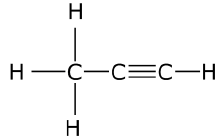2.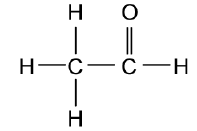(ii) What is the special feature of the structure of ethyne?

(iii) Name the saturated hydrocarbon containing two carbon atoms.

(iv) Give the structural formula of acetic acid.

(g) Give the appropriate term defined by the statements given below:

(i) The formula that represents the simplest ratio of the various elements present in one molecule of the compound.

(ii) The substance that releases hydronium ion as the only positive ion when dissolved in water.

(iii) The tendency of an atom to attract electrons towards itself when combined in a covalent compound.

(iv) The process by which certain ores, specially carbonates, are converted to oxides in the absence of air.

(v) The covalent bond in which the electrons are shared equally between the combining atoms.

(h) Arrange the following according to the instructions given in brackets:

(i) K, Pb, Ca, Zn (In the increasing order of reactivity)

(ii) Mg2+, Cu2+, Na1+, H1+ (In the order of preferential discharge at the cathode)

(iii) Li, K, Na, H (In the decreasing order of their ionisation potential)

(iv) F, B, N, O (In the increasing order of electron affinity)

(v) Ethane, methane, ethene, ethyne (In the increasing order of molecular weight) [H = 1, C = 12]

Question 2

(a) Draw the electron dot structure of

(i) Nitrogen molecule [N = 7]

(ii) Sodium chloride [Na = 11, Cl = 17]

(iii) Ammonium ion [N = 7, H = 1]

(b) The pH values of three solutions A, B and C are given in the table. Answer the following questions: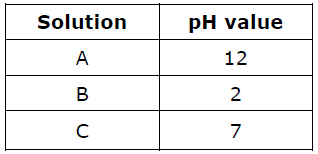(i) Which solution will have no effect on litmus solution?

(ii) Which solution will liberate CO2 when reacted with sodium carbonate?

(iii) Which solution will turn red litmus solution blue?

(c) Study the extract of the periodic table given below and answer the questions that follow. Give the letter corresponding to the element in question.

DO NOT repeat an element.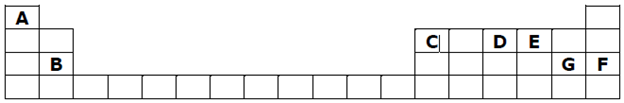(i) Which element forms an electrovalent compound with G?

(ii) The ion of which element will migrate towards the cathode during electrolysis?

(iii) Which non-metallic element has the valency of 2?

(iv) Which is an inert gas?

Question 3

(a) Name the particles present in

(i) Strong electrolyte

(ii) Non-electrolyte

(iii) Weak electrolyte

(b) Distinguish between the following pairs of compounds using the reagent given in the brackets.

(i) Manganese dioxide and copper (II) oxide (using concentrated HCl)

(ii) Ferrous sulphate solution and ferric sulphate solution (using sodium hydroxide solution)

(iii) Dilute hydrochloric acid and dilute sulphuric acid (using lead nitrate solution)

(c) Choose the method of preparation of the following salts from the methods given in the list:

[List:   A. Neutralisation                   B. Precipitation

C. Direct combination          D. Substitution]

(ii) Iron (II) sulphate

(iii) Sodium nitrate

(iv) Iron (III) chloride

Question 4

(a) Complete the following equations:

(i) S + conc. HNO3

(ii) C + conc. H2SO4

(iii) Cu + dil. HNO3

(b) Write a balanced chemical equation for the preparation of

(i) Ethene from bromoethane

(ii) Ethyne using calcium carbide

(iii) Methane from sodium acetate

(c) Name the following organic compounds:

(i) The compound with 3 carbon atoms whose functional group is carboxyl.

(ii) The first homologue whose general formula is CnH2n.

(iii) The compound that reacts with acetic acid to form ethyl ethanoate.

(iv) The compound formed by complete chlorination of ethyne.

Question 5

(a) Give the chemical formula of

(i) Bauxite

(ii) Cryolite

(iii) Sodium aluminate

(b) Answer the following question based on the extraction of aluminium from alumina by Hall-Heroult’s process:

(i) What is the function of cryolite used along with alumina as the electrolyte?

(ii) Why is powdered coke sprinkled on top of the electrolyte?

(iii) Name the electrode from which aluminium is collected.

(c) Match the alloys given in column I to the uses given in column II.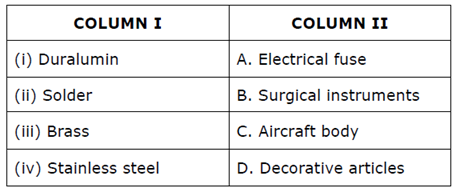Question 6

(a) Identify the substances underlined:

(i) The catalyst used to oxidise ammonia.

(ii) The organic compound which when solidified forms an ice-like mass.

(iii) The dilute acid which is an oxidising agent.

(b) Copper sulphate solution reacts with sodium hydroxide solution to form a precipitate of copper hydroxide according to the equation:

2NaOH + CuSO4 → Na2SO4 + Cu(OH)2

(i) What mass of copper hydroxide is precipitated by using 200 gm of sodium hydroxide?

[H = 1, O = 16, Na = 23, S = 32, Cu = 64]

(ii) What is the colour of the precipitate formed?

(c) Find the empirical formula and the molecular formula of an organic compound from the data given below:

C = 75.92%, H = 6.32% and N = 17.76%

The vapour density of the compound is 39.5.

[C = 12, H = 1, N = 14]

Question 7

(a) Name the gas evolved in each of the following cases.

(i) Alumina undergoes electrolytic reduction.

(ii) Ethene undergoes hydrogenation reaction.

(iii) Ammonia reacts with heated copper oxide.

(b) Study the flow chart given and give balanced equations to represent the reactions A, B and C: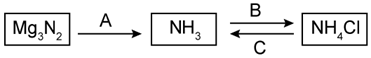(c) Copy and complete the following table which refers to the industrial method for the preparation of ammonia and sulphuric acid: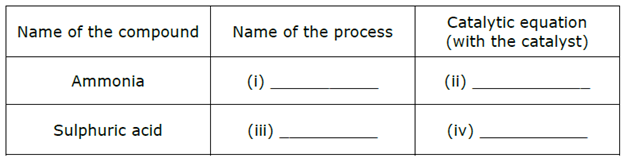## Key Features of Study Materials for ICSE Class 10 Chemistry:

• According to the latest ICSE syllabus
• Prepared by subject matter experts